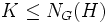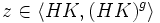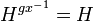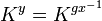# Pronormality is normalizing join-closed

This article gives the statement, and possibly proof, of a subgroup property (i.e., pronormal subgroup) satisfying a subgroup metaproperty (i.e., normalizing join-closed subgroup property)
View all subgroup metaproperty satisfactions | View all subgroup metaproperty dissatisfactions |Get help on looking up metaproperty (dis)satisfactions for subgroup properties
Get more facts about pronormal subgroup |Get facts that use property satisfaction of pronormal subgroup | Get facts that use property satisfaction of pronormal subgroup|Get more facts about normalizing join-closed subgroup property

## Statement

Suppose$H, K \le G$ are pronormal subgroups such that$K \le N_G(H)$: in other words,$K$ normalizes$H$. Then the join of subgroups$\langle H, K \rangle$ (also the same as$HK$) is also a pronormal subgroup.

## Related facts

### Similar facts: statements about normalizing join-closedness

Some facts with very similar proofs:

Other facts about normalizing joins, but with a different kind of proof:

## Proof

This proof uses a tabular format for presentation. Provide feedback on tabular proof formats in a survey (opens in new window/tab) | Learn more about tabular proof formats|View all pages on facts with proofs in tabular format
CONVENTION WARNING: This article/section uses the right-action convention. The left and right action conventions are equally powerful and statements/reasoning here can be converted to the alternative convention (the main reason being that every group is naturally isomorphic to its opposite group via the inverse map). For more on the action conventions and switching between them, refer to switching between the left and right action conventions.

Given: A group$G$, pronormal subgroups$H,K \le G$ such that$K \le N_G(H)$.

To prove:$HK$ is pronormal in$G$. Specifically, for any$g \in G$, our goal is to find a$z \in \langle HK, (HK)^g \rangle$ such that$(HK)^z = (HK)^g$.

Proof: The overall problem in showing that there exists such an element$z$ is to find something that simultaneously works for both$H$ and$K$ for the same element$g$. The solution to that is to first find an element (here called$x$) that works for$H$. This original element$x$ need not work for$K$. However, since$K$ normalizes$H$, we can now tweak this element to fix the behavior on$K$ without altering the behavior on$H$. The tweaking happens via multiplication by a suitable element in$N_G(H)$.

The proof has the flavor of constructing an upper triangular transformation.

Step no. Assertion/construction Given data used Previous steps used Explanation Commentary
1 There exists$x \in \langle H, H^g \rangle$ such that$H^x = H^g$.$H$ is pronormal in$G$,$g \in G$. From definition of pronormal. Taken care of$H$ using the element$x$, but no control over whether$K$ goes where it should.
2$\! H^{gx^{-1}} = H$ Step (1) [SHOW MORE] Moving to a corresponding element that stabilizes/normalizes$H$. This reference element$gx^{-1}$ will be the model for the tweak element$y$ that we'll construct soon.
3$\langle K, K^{gx^{-1}} \rangle \le N_G(H)$$K$ normalizes$H$, i.e.,$K \le N_G(H)$ Step (2) [SHOW MORE] We capitalize on the fact that$K$ normalizes$H$ in order to move inside$N_G(H)$.
4 There exists$y \in \langle K, K^{gx^{-1}} \rangle$ such that$\! K^y = K^{gx^{-1}}$.$K$ is pronormal in$G$ From definition of pronormal. We find the tweak element$y$ that fixes$K$.
5 In particular,$y$ obtained in Step (4) is in$N_G(H)$, so$H^y = H$. Steps (3), (4) Follows directly by combining steps. We make sure that$y$ has no effect on$H$.
6 Consider$z = yx$. Then$\! (HK)^z = (HK)^x$ Steps (1), (4), (5) [SHOW MORE] We combine the elements$y$ and$x$ to make sure that both$H$ and$K$, and hence$HK$, land where needed.
7$z \in \langle (HK), (HK)^g \rangle$ Steps (1)--(6) [SHOW MORE] We check that the element$z$ thus constructed is inside the required join.

Steps (6) and (7) clinch the proof.